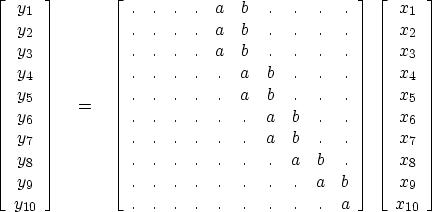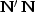Next: DERIVATIVE AND INTEGRAL Up: NORMAL MOVEOUT AND OTHER Previous: Null space and inconsistency

## NMO with linear interpolation

NMO with linear interpolation implies that the matrixis a two-band matrix. Each row has exactly two elements that interpolate between two elements on the input. I will sketch the appearance of the matrix, using the letters a and b for the elements. Each a and b is different numerically, but on a given row, a+b=1.(21)

Here the matrixis tridiagonal, but I am going to let you work out the details by yourself. The original data can be recovered by solving the tridiagonal system. This method can be used to program an invertible NMO or to program an invertible trace interpolation. I do not want to clutter this book with the many details. Instead, I present spot1(), a convenient subroutine for linear interpolation that can be used in many applications.

# Nearest neighbor interpolation would do this:    val = vec( 1.5 + (t-t0)/dt)
# This is the same but with _linear_ interpolation.
#
real tc, fraction,             t0,dt, t, val,    vec(nt)
tc      = (t-t0) / dt
itc     =  tc
it      = 1 + itc;                      fraction = tc - itc
if( 1 <= it  &&  it < nt)